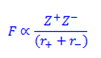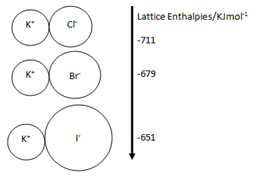# Chemistry A2

?
• Created by: Davina
• Created on: 03-10-12 11:03

## Entropy, S

• Entropy, S, is a measure of 'disorder' of a system
• Entropy is a measure to the extent to which energy is dispersed.
• Substances with high entropy are nearly alike than those with low entropy.
• Entropy tends to increase in natural processes.
• disorder tends to increase
• energy tends to become more dispersed
• things that are different tend to become less distinguishable
• It is a thermochemical quantity that makes it possible to decide whether or not a reaction is spontaneous.
• A system becomes energetically stable when it becomes more disordered.
• Ludwig Boltzmann derived the formula S = k ln W
1 of 13

## Entropy, Different States

• Solids have relatively low  values for standard molar entropies. The atoms/molecules are fixated in place.
• In diamond, carbon atoms are held in place by strong directional covalent bonds. Lead has a higher value for standard molar entropy, because metallic bonds are not directional . The  heavier and larger lead atoms, can vibrate more  freely and share out  energy in more ways that diamond.
• Liquids have higher standard molar entropies than solids because the atoms/molecules are free to move. There are many more ways in distributing the particles and energy.
• Standard molar entropy of mercury is greater than lead. Molecules with more atoms have higher standard entropies because they can vibrate, rotate and share out energy in more ways.
• Gases have higher standard molar entropies than liquids, the atoms/molecules not only are free to move, but are widely spaced. There are even more ways of distributing the particles energy.
• Entropy of argon is even higher than mercury. As with liquids, molecules with more atoms can rotate, vibrate and arrange themselves in more ways.
2 of 13

## Entropy, Calculations I

Entropy change of the system

ΔS system = ΣΔS products - ΣΔS reactants

• If ΔS system is positive, there is an increase in disorder.
• If ΔS system is negative, there is a decrease in disorder.
• Example: Calculate the entropy change of ΔS system, for the reaction:

2Mg (s) + O2 (g) → 2MgO (s)         [MgO = 26.9], [O2 = 102.5], [Mg = 32.7]

ΔS system = ΣΔS products - ΣΔS reactants

ΔS system  = [2(26.9)] - [2(32.7) + 102.5]

= - 114.1 JK-1mol-1

• This shows that entropy of system decreases when nitrogen and hydrogen react to form ammonia. Half the no. of gas molecules in the products than  in reactants.
• This is expected since, the no. of possible arrangements of molecules has reduced.
3 of 13

## Entropy, Calculations II

Entropy change of surroundings

ΔSsurroundings = -ΔH/T       NOTE: T in kelvin and ΔH is in joules

• The minus sign is included, as the entropy charge is larger as more heat is released in surroundings. For an exothermic reaction ΔH is negative and so -ΔH is positive.
• For any value of heat energy, the increase in entropy in the surrounding is greater when surroundings are cool, than when they are hot.
• There's greater effect on the no. of ways of distributing energy when adding heat to atoms in cold system, than adding same amount of heat energy to a already hot system.
• Example: Consider the reaction, under standard conditions

2Mg (s) + O2 (g) → 2MgO (s)  ΔH= - 1204 KJmol-1

ΔSsurroundings = -ΔH/T  = -(-1204000)/298

=+ 4040 JK-1mol-1

4 of 13

## Entropy, Calculations III

Total entropy change

ΔStotal = ΔS system + ΔS surroundings

• For changes that happen spontaneously, ΔS must be positive. Changes that tend to proceed naturally are called spontaneous changes, they can be quite slow.
• Example: Calculate total entropy change of:

2Mg (s) + O2 (g) → 2MgO (s)  ΔS sys= - 114.1JK-1mol-1 , ΔS sur = +4040JK-1mol-1

ΔStotal = ΔS system + ΔS surroundings = [-114.1] + [+4040] = 3925.9

= 3930 JK-1mol-1 (3 s.f.)

• Most exothermic reactions tend to go as room temperature because the value -ΔH/T  is much larger and more positive than ΔS system, which means ΔStotal is positive
• An endothermic reaction can be spontaneous if ΔS system >ΔS surroundings.
• A reaction that tends not to go a room temperature may become spontaneous as the temperature rises because ΔS surroundings decreases as T increases.
5 of 13

## Lattice Enthalpy, Theoretical Lattice

• Theoretical lattice can be calculated from the expression that relates the force of attraction F, between the ions to the size and charge of ions:• Where:

Z+ = no. of charge on cation

Z- = n. of charge on anion

6 of 13

## Lattice Enthalpy, Ionic Lattices

• Stronger the electrostatic attraction between oppositely charged ions the more exothermic.
• Small ions pack together closely in an ionic lattice and attract each other strongly.
• Larger the size, the further apart in the ionic lattice and the electrostatic forces of attraction are weaker.7 of 13

## Lattice Enthalpy, Ionic Lattices II

• As the size of the charge on the ions increases, the lattice enthalpy becomes more exothermic, as there is a stronger electrostatic force attraction between the ions.
• Compounds with the most negative lattice enthalpies are those that have small, highly charged ions.

(insert - hmmm)

• As charge increases, the ions decreases in ionic radius produce a greater attraction between these positive and negative ions.
• Decrease in ionic radius brings the ions in lattice closer together, producing stonger electrostatic attraction.
8 of 13

## Lattice Enthalpy, Theoretical Values

• Experimental lattice enthalpies are the value s of lattice enthalp calculated from Born-Harber cycles.
• Theoretical values are calculated on the basis of a purely ionic model - 100% ionic character exists in the compound or ions are perfectly spherical.
• If there is good agreement between the theoretical values and experimental value, then the pure ionic model of the bonding compound is an accurate one.
• However, if there is poor agreement between these two values, then the compound has a slightly covalent character, in addition to ionic bonding present.
• Example: Silver cholride, Experimental = -890 KJmol-1 , Theoretical = -769 KJmol-1

Insert Immm 2nd

• Small highly polarising Ag+ distorts the electron cloud of Cl-, thereby introducing covalent character into the bond
• The experimental lattice enthalpy above is -121 KJmol-1 more exothermic than theoretical value. It shows the lattice has been strengthened by a degree of covalency in the bonding.
9 of 13

## Lattice Enthalpy, Fajans' rules

• Extent of polarisation of the negative ion by the positive ion is described by Fajan's rule.
• There is a tendancy towards increasing covalent character in an ionic compound when:
• the positive ion is small
• the posiive ion has multiple positive charges
• the negative ion is large
• the negative ion has multiple negative charges

insert hmmm 3rd

• Calculated valuesof standard enthalpy change can be used to predict the stoichiometry of an ionic compound.
10 of 13

## Group 2, Metals I

Physical Properties

• It is seen that 1st IE decreases down group 2 (2nd IE follows trend)
• i.e. It is becoming progressively easier to remove outer electrons
• The atomic radius increases down the group as more shells are added.
• atomic number increases down the group
•
• Ionisation energy decreases because ...
• ...although nuclear charge increases, shielding from increasing number of e- shells down the group cancels out the additional problems.
• ...as atomic radius increases, the outer e- is becoming further away from the nucleus, experiences a weaker force of attraction.
• ...thus means that the outer e-s becomes progressively easier to remove, therefore IE decreases down the group.

11 of 13

## Group 2, Metals II

Hydroxides

• Mg(OH)2
• Ca(OH)2
• Sr(OH)2
• Ba(OH)2
• Solubility increases down the group
• pH of solution increases down a group

Carbonates + Nitrates

• MgCO2                                       Mg(NO3)2
• CaCO2                                       Ca(NO3)2
• SrCO2                                         Sr(NO3)2
• BaCO2                                       Ba(NO3)2
• Decreasing ease of thermol decomposition down a group
12 of 13

## Group 2, Metals III

Summary reactions of Group 2

insert image .....

13 of 13